# 玉玲珑技能链

2021-02-01更新

uboy才没有小脸圆圆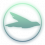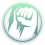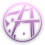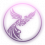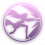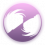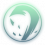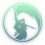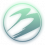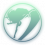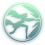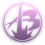## 添加技能链

```{{技能链
|技能链名称=标准心法模板（复制用）
|适用等级=
|职业名称=
|上链心法1=
|上链副心法1=
|上链技能1=
|上链心法2=
|上链副心法2=
|上链技能2=
|上链心法3=
|上链副心法3=
|上链技能3=
|上链心法4=
|上链副心法4=
|上链技能4=
|上链心法5=
|上链副心法5=
|上链技能5=
|上链心法6=
|上链副心法6=
|上链技能6=
|下链心法1=
|下链副心法1=
|下链技能1=
|下链心法2=
|下链副心法2=
|下链技能2=
|下链心法3=
|下链副心法3=
|下链技能3=
|下链心法4=
|下链副心法4=
|下链技能4=
|下链心法5=
|下链副心法5=
|下链技能5=
|下链心法6=
|下链副心法6=
|下链技能6=
|技能链描述=
}}
<!-- 技能链结束 -->
```